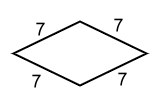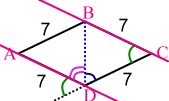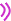### Are these really parallelograms - answers

These are answers to my earlier post where I asked if certain figures necessarily are parallelograms.

The question was: Does the given information in each diagram guarantee that each is a parallelogram?

Figure 1:This one you can't get around; it ends up being a parallelogram, actually a dandy rhombus. Let's prove it. You can notice it has lots of sides of the same length. If we draw a diagonal, we get two triangles with all kinds of same sides:The two triangles ABD and BCD end up having all three sides the same. So by the SSS triangle congruence theorem, they are congruent triangles. Hence, their corresponding angles are the same.

I've marked the corresponding angles with the same colors. Actually the triangles are even isosceles so the blue and purple angles are even congruent... but we don't need that fact.

To prove ABCD is a parallelogram, we need to prove its two sides are parallel. And for that, it's often handy to use the corresponding angle theorem: if corresponding angles are equal, the lines are parallel. So image that we continue the line segment CD. Notice the additional green angle that I've marked:How do I know it actually is a "green angle" (congruent with the other green angle)? It's because the three angles, being angles of a triangle, add up to 180:++= 180. And the three angles being there along the same line (the continuation of CD), it must be. This sounds a little too complicated as I'm typing it. Perhaps I shouldn't have marked it green. Anyhow, since it IS congruent with the other green angle at C, then the line segments BC and AD must be parallel.

A similar argument would prove the other two sides parallel.

Figure 2:This isn't necessarily a parallelogram, but it IS always a trapezoid:Figure 3:This one is trickier, but it isn't necessarily a parallelogram. I used a compass to find a way to make this into a trapezoid:ABCD is a trapezoid with the non-parallel sides 5 units long.

Figure 4:This one is actually a repetition of the Figure 1, because it has the opposites sides of same length. We can use the identical argument to prove it is a parallelogram.